Jekyll2022-08-11T10:57:17+00:00www.dariosanfilippo.com/feed.xmlblankA simple website for an algorithmic music researcher. Efficient exponential interpolation using one-pole filters2022-08-09T00:00:00+00:002022-08-09T00:00:00+00:00www.dariosanfilippo.com/blog/2022/efficient_exponential_interpolationExponential curves are particularly useful in digital audio as they provide natural-sounding amplitude variations, and they describe the behaviour of dis/charging capacitors in analogue RC circuits [Chamberline 1985]. A common task, among others, is modelling analogue ADSR envelope generators, which are based on such circuits.

Digital one-pole filters can emulate these circuits, although they would require infinite time to reach the target value exactly. Nigel Redmon from Earlevel Engineering has addressed this problem using overshooting one-pole filters, which he discusses in a series of blog posts. Here, we will see a solution following the same principle but with a slightly different formalisation that turns one-pole filters into an exponential mapping function with arbitrary dis/charging rates passing through two arbitrary values. This is useful as it allows computing the exponential interpolation using one multiply and two sums per sample rather than one exponential function and one multiply.

Given start and end values, respectively, $$y_0$$ and $$y_1$$, there is an infinite family of exponential mapping functions connecting these two points:

$\begin{equation} f(x) = \frac{1-e^{-kx}}{1-e^{-k}}(y_1 - y_0) + y_0 \label{expInter} \end{equation}$

where $$x \in [0..1]$$ is a real interpolation index, and $$k \neq 0$$ is a parameter determining the dis/charging rate. For values of $$k$$ approaching $$0$$, the exponential function approximates linear interpolation, whereas values further away from 0 will increase the tension of the curve in either directions. See this Desmos graph for a visual representation.

A digital one-pole filter has the form:

$\begin{equation} y[n] = x[n] + \alpha (y[n-1] - x[n]) \end{equation}$

where the feedback coefficient is given by:

$\begin{equation} \alpha = e^{(-kT)/t} \end{equation}$

where $$T$$ is the sampling period, and $$t$$ is the filter period. In other words, the step-response of the filter will reach $$1-e^{-k}$$ in the given time $$t$$.

Similarly to how we normalise the output of Eq. \ref{expInter}, we can adjust the input and initial state of the filter to exponentially connect starting and ending values:

$y[n] = \begin{cases} y_0 & \text{if } n = 0 \\ x[n] + \alpha (y[n-1]-x[n]) & \text{if } n > 0 \end{cases}$

by setting the input of the filter to the value:

$\begin{equation} x[n] = \frac{y_1 - y_0}{1-e^{-k}} + y_0 \quad . \end{equation}$

The output of the filter closest to the target value is after $$\lfloor t / T \rceil + 1$$ samples, hence we can adjust the filter state and coefficient at the end of each segment, while the computation throughout the segment only requires one multiply and two additions. Comparing the one-pole interpolation with the exponantial mapping using std::exp() in C++, we have a mean square error equal to $$3.777937251925323E-7$$ in single-precision processing.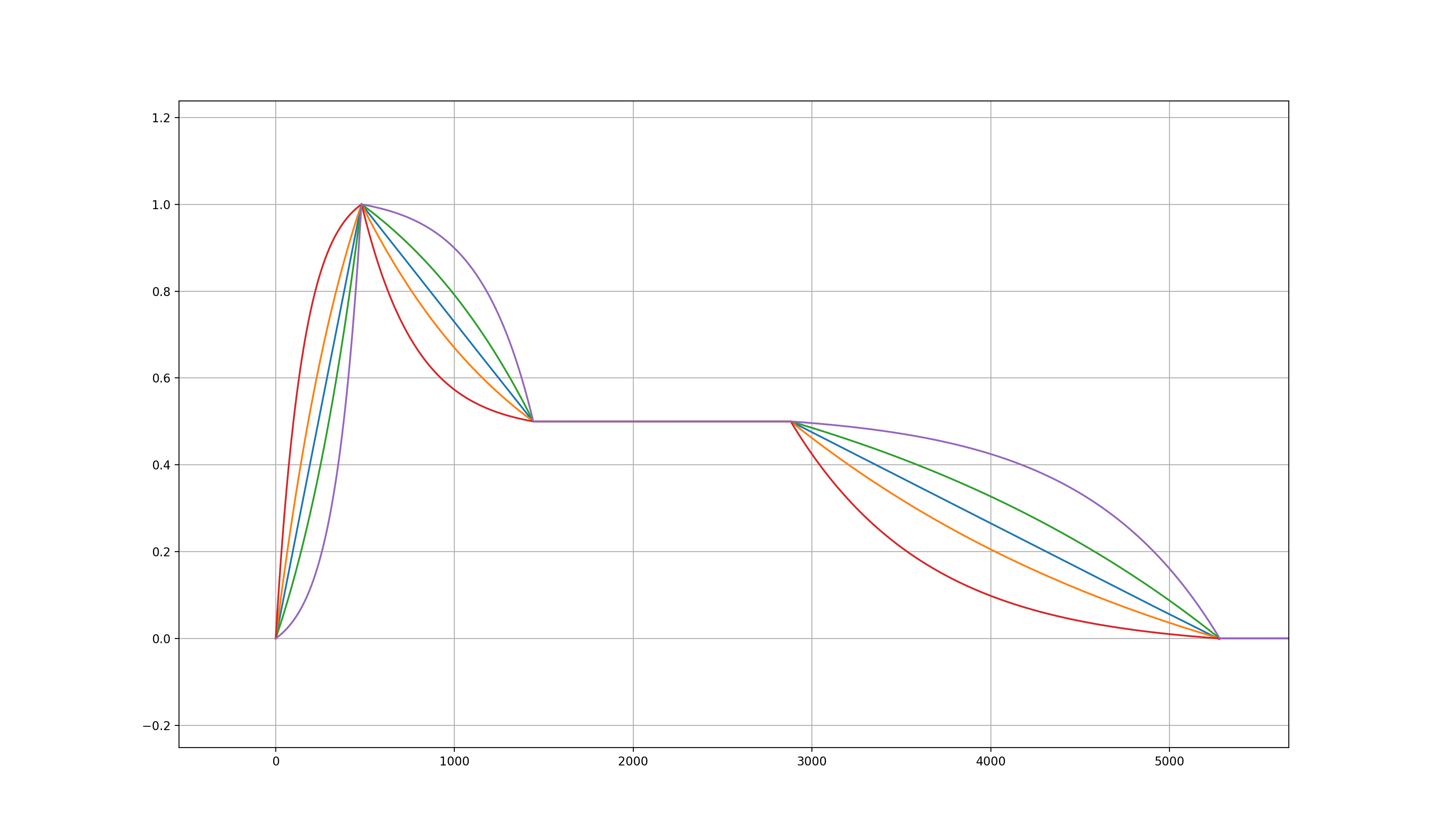ADSR generation using one-pole filters and different values of k.
]]>
Handling infinity and not-a-number (NaN) values in Faust and C++ audio programming2020-12-28T00:00:00+00:002020-12-28T00:00:00+00:00www.dariosanfilippo.com/blog/2020/handling_inf_nan_values_in_faust_and_cppFaust is a high-level programming language for audio based on C++ where several implementation-related low-level issues are handled by the Faust compiler. However, Faust still allows for a certain degree of freedom in its operations that may result in undesired behaviours for the domain of audio streams. This blog post shows a few examples where the special values of $$\mathit{inf}$$ and $$NaN$$ can be generated and how to avoid that. As we will see, $$\mathit{inf}$$ and $$NaN$$ values are problematic for audio streams as they follow peculiar arithmetic and relational rules.

$$\mathit{Inf}$$, both positive or negative, is a special value that is used to represent an exceedingly large number, namely a value that is too large to be represented accurately by the floating-point type used. For example, exponential and unbounded growths can occur in unstable recursive systems, which eventually result in $$\mathit{inf}$$ or $$\mathit{-inf}$$. Also, in recursive systems, the opposite case, that is an exponential decay where a value decreases and tends towards 0, can still be a problem when reaching the representation limits of the data type for small values. These values are commonly called subnormal values, and they can be CPU-intensive. Luckily, efficient flush-to-zero (FTZ) mechanisms are usually deployed at the hardware-level to overcome the problem.

$$\mathit{Inf}$$ or $$\mathit{-inf}$$ can also be generated through the multiplication of very large values, or by the division between a large value and a small enough one. Below, we can summarise the arithmetic of $$\mathit{inf}$$ values, alone or combined with real numbers, through the output of common operators and functions in Faust and C++. These examples also show how indeterminate forms are handled.

\begin{align*} & \infty \cdot x = \infty \cdot sgn(x) \quad \{x \in \mathbb{R} : x \neq 0\} \\ & \infty \cdot (\pm \infty) = \pm \infty \\ & \pm \infty \cdot 0 = NaN \\ & \infty / x = \infty \cdot sgn(x) \quad \{x \in \mathbb{R} : x \neq 0\} \\ & \pm \infty / (\pm \infty) = NaN \\ & \pm \infty / 0 = \pm \infty \\ & 0 / 0 = NaN \\ & x \bmod 0 = NaN \quad \{x \in \mathbb{R}\} \\ & \pm \infty \bmod x = NaN \quad \{x \in \mathbb{R}\} \\ & \pm \infty + x = \pm \infty \quad \{x \in \mathbb{R}\} \\ & \pm \infty - x = \pm \infty \quad \{x \in \mathbb{R}\} \\ & \pm \infty \pm \infty = \pm \infty \\ & \infty - \infty = NaN \\ & \pm \infty^0 = 1 \\ & \pm \infty^1 = \pm \infty \\ & \pm \infty^{-1} = \pm 0 \\ & \pm \infty^{\infty} = \infty \\ & \pm \infty^{-\infty} = 0 \\ & \pm 1^{\pm \infty} = 1 \\ & 0^0 = 1 \\ & \sqrt{x} = NaN \quad \{x \in \mathbb{R} : x < 0\} \\ & \sqrt{\infty} = \infty \\ & \sqrt{-\infty} = NaN \\ & \log(0) = -\infty \\ & \log(\infty) = \infty \\ & \log(x) = NaN \quad \{x \in \mathbb{R} : x < 0\} \\ & cos(\pm \infty) = NaN \\ & sin(\pm \infty) = NaN \\ & tan(\pm \infty) = NaN \\ & acos(\pm \infty) = NaN \\ & acos(x) = NaN \quad \{x \in \mathbb{R} : x < -1 \lor x > 1\} \\ & asin(\pm \infty) = NaN \\ & asin(x) = NaN \quad \{x \in \mathbb{R} : x < -1 \lor x > 1\} \\ & atan(\pm \infty) = \pm \pi / 2 \\ & acosh(x) = NaN \quad \{x \in \mathbb{R} : x < 1\} \\ & acosh(\infty) = \infty \\ & acosh(-\infty) = NaN \\ & asinh(\pm \infty) = \pm \infty \\ & atanh(\pm 1) = \pm \infty \\ & atanh(x) = NaN \quad \{x \in \mathbb{R} : x < -1 \lor x > 1\} \\ & cosh(\pm \infty) = \infty \\ & sinh(\pm \infty) = \pm \infty \\ & tanh(\pm \infty) = \pm 1 \\ \end{align*}

We can see that several of these operations produce $$NaN$$, which is an even more problematic value for audio. Particularly, any operation where one of the operands is $$NaN$$ produces a $$NaN$$, and any relational operation containing a $$NaN$$ is false. Thus, the possibility of a $$NaN$$ value contaminating the audio stream may result in a chain reaction where these values spread really fast. If a $$NaN$$ value is used to access an array cell, for example, in a delay line, the program is likely to end with a segmentation fault error. Thus, it is vital to prevent $$NaNs$$ to enter audio streams, as well as to prevent $$\mathit{inf}$$ values since they, too, can result in $$NaNs$$. Also, note that signed zeroes are important for FTZ mechanisms, as we can at least preserve the sign of the subnormal value. See [Goldberg 1991] for more.

C++ provides useful values for representable limits in the limits library. The std::numeric_limits::max(), std::numeric_limits::min(), and std::numeric_limits::epsilon() functions output constants representing, respectively, the largest and smallest representable values, and the relative rounding error such that $$\epsilon$$ is the smallest quantity for which $$1 + \epsilon > 1$$. In double precision, these are:

\begin{align*} & 1.7976931348623158e+308 \\ & 2.2250738585072014e-308 \\ & 2.2204460492503131e-016 \end{align*}

The constant $$\epsilon$$ can be useful when some variable must be set to a value just below $$1$$, for example, when we need the pole of a filter as close as possible to the unit circle. The constant $$MIN$$ can be used as a limit to avoid division by $$0$$. For example, a safe division operator in Faust can be implemented as follows:

The output of the division $$1/0$$ using the safe_div function is $$4.4942328371557898e+307$$. It is very close to the $$MAX$$ constant, meaning that it can easily become $$\mathit{inf}$$. Alternatively, the $$\epsilon$$ constant can be used as a limit as in:

In this case, the output of $$1/0$$ is $$4503599627370496$$, which is in a much safer range at the expenses of accuracy for some divisions. Still, if the numerator were greater than $$MAX \cdot \epsilon$$, it would become $$\mathit{inf}$$. Otherwise, we could simply clip the output of the $$/$$ operator to $$MAX$$ and $$-MAX$$ to guarantee that neither $$\mathit{inf}$$ or $$NaN$$ values are output:

Note that $$\mathit{ma.INFINITY}$$ corresponds to $$MAX$$ in Faust, and that we must delay the value that defines the denominator; otherwise, the Faust compiler will detect a division by zero and will fail to compile. For the denominator, we generated a $$-0$$ at the second sample, which results in the $$-MAX$$ constant. Also note that this division is effective to keep audio streams clean from $$NaN$$ and $$\mathit{inf}$$ values, although the division by zero can still take place. However, if the hardware follows the IEEE 754 standard, the floating-point division by zero will produce $$\pm \mathit{inf}$$ instead of being signalled as an exception by the C++ program, unless both numerator and denominator are $$0$$, in which case it will produce $$NaN$$. For the same reason, consider that Faust’s syntax is strict and that all branches of an if-statement are always evaluated; you may want to read this as well.

Another problem of clipping only the output of a function as a guard is that $$NaN$$ values are never output in std::max() and std::min() functions, hence whether the indeterminate form $$0/0$$ results in $$MAX$$ or $$MIN$$ only depends on the implementation of the clipping function, namely whether we first check against the upper or lower limit. In general, the most effective guards that we have against $$NaN$$ and $$\mathit{inf}$$ values are the std::max() and std::min() functions combined with the numerical limit constants above to effectively limit the domain of other functions. If an operator or function is indeterminate for some input, then it is necessary to limit the input domain and, in some cases, the output domain too. Otherwise, limiting only the output is adequate. A strict safe division function in Faust could then look like this:

In this case, the output of $$0$$ divided by any real value including $$0$$ is always $$0$$. A safe std::sinh() function, instead, can be defined as follows, which will guarantee real values between $$MAX$$ and $$-MAX$$ just by clipping its output:

For the std::log() function, for example, the input domain could be limited to $$MIN$$ and $$MAX$$, giving a rather safe output domain between $$-708.39641853226408$$ and $$709.78271289338397$$:

Alternatively, the output domain can be clipped to $$-MAX$$ and $$MAX$$, making sure that we first check against the lower bound so that $$NaN$$ values generated by negative inputs are clipped to $$-MAX$$:

Finally, I would like to thank my brother, Salvatore Sanfilippo, and Oli Larkin for a few valuable comments on this post.

]]>
Three ways to implement recursive circuits in the Faust language2020-11-28T00:00:00+00:002020-11-28T00:00:00+00:00www.dariosanfilippo.com/blog/2020/faust_recursive_circuitsAfter a long silence, this post is about the implementation of not-so-simple recursive circuits in the Faust language. We will see a few relevant examples and the three main approaches that we can follow to implement a circuit: Faust’s diagram expressions (basic syntaxt), the with environment with auxiliary function definition, and the letrec environment.

The Faust manual provides basic examples for the first, second, and third approaches. As we will see later, Faust’s basic syntax can be less concise and more complicated in some cases, whereas the remaining two approaches are easier. However, the letrec environment, despite being concise, is not always desirable if we want to generate diagrams that have little or no redundancy. In this post, we will implement a few circuits with feedback using all of the three approaches.

Let’s start with a simple one-pole lowpass filter, which is essentially a scaled-down input feeding into an integrator. In the basic syntax, the tilde operator lets the signal(s) to its left through and sendsthem back into a feedback path to fill the first available input(s) in the function. The operand or group of operands immediately after the tilde operator is applied to the feedback path. The tilde operator, unlike all other basic synthax operators, is left-assiociative and has highest priority. For example, if we write:

we are summing the first two inputs, then we are sending the result together with a third input into another “+” operator, and then we are summing the result to the output itself. Of course, any feedback loop in a digital system requires at least a one-sample delay, which is the default delay in Faust’s recursive composition. Suppose that we want to add another feedback loop in the previous function that is connected to the input of the first “+” operator. We also want to multiply that feedback signal by .5. Then we can write as follows:

Back to the lowpass filter, we can see the diagram below, kindly taken from the website of Julius Smith.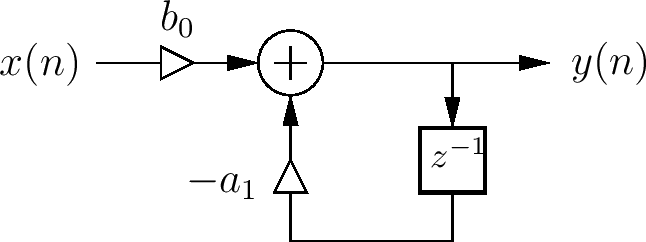Following [Chamberlin 1985] for the design of the filter, we can write the function using basic syntax as follows:

Below, we can see the diagram generated by the Faust code. Note that the empty little square on a wire indicates a one-sample delay, representing the $$z^-1$$ operator in our case.Block diagram generated through basic syntax.

Another way to implement the filter is by using an intermediate function and the with environment. I would also like to thank Oleg Nesterov who first introduced me to this technique. The intermediate function usually acts as container and defines elementary single or multiple feedback loops. The feedback loops that are sent back to the function can then be used anywhere in the inner code as they are identified by argument names, which are specified in the intermediate function (“loop”) definition: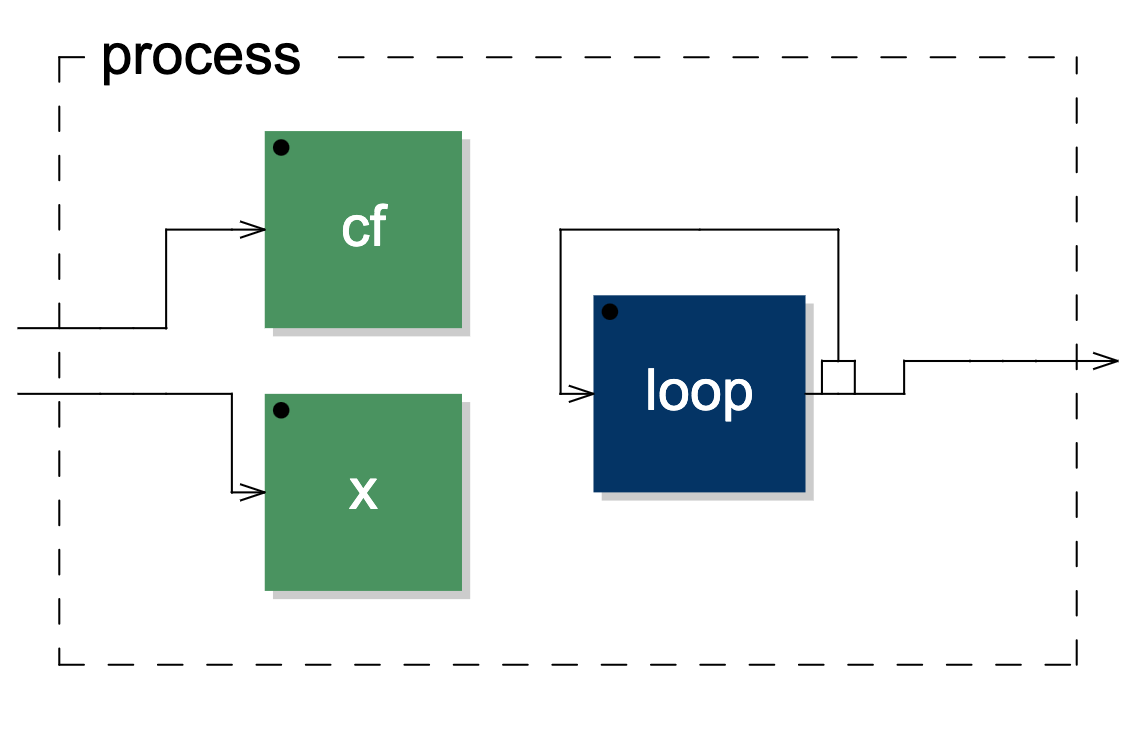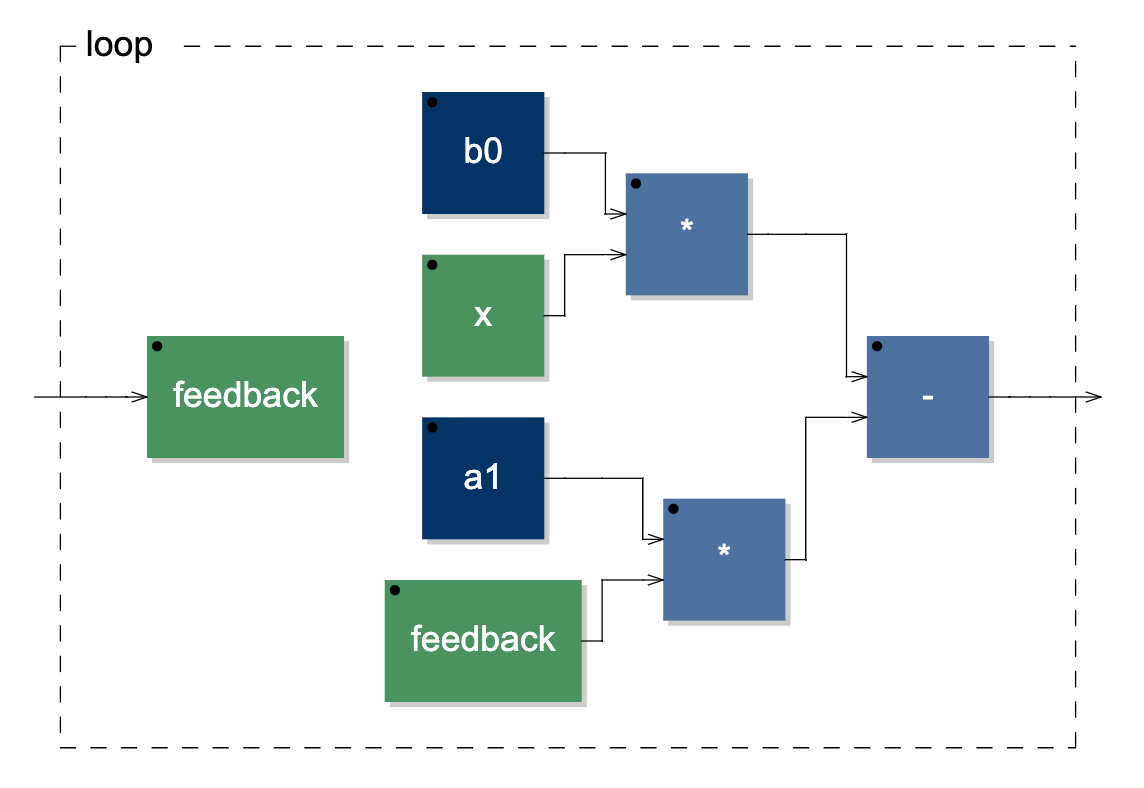Block diagram generated through intermediate function and the with environment.

The third way is through the letrec environment. Within this environment, we can define signals recursively, similarly to how recurrence equations are written: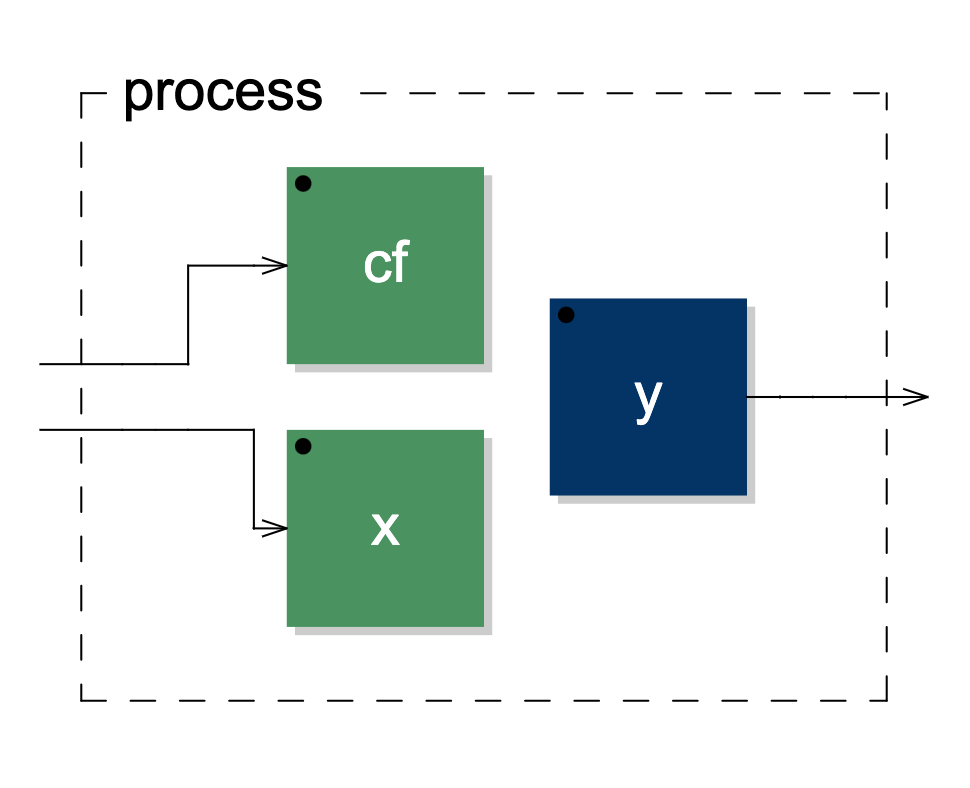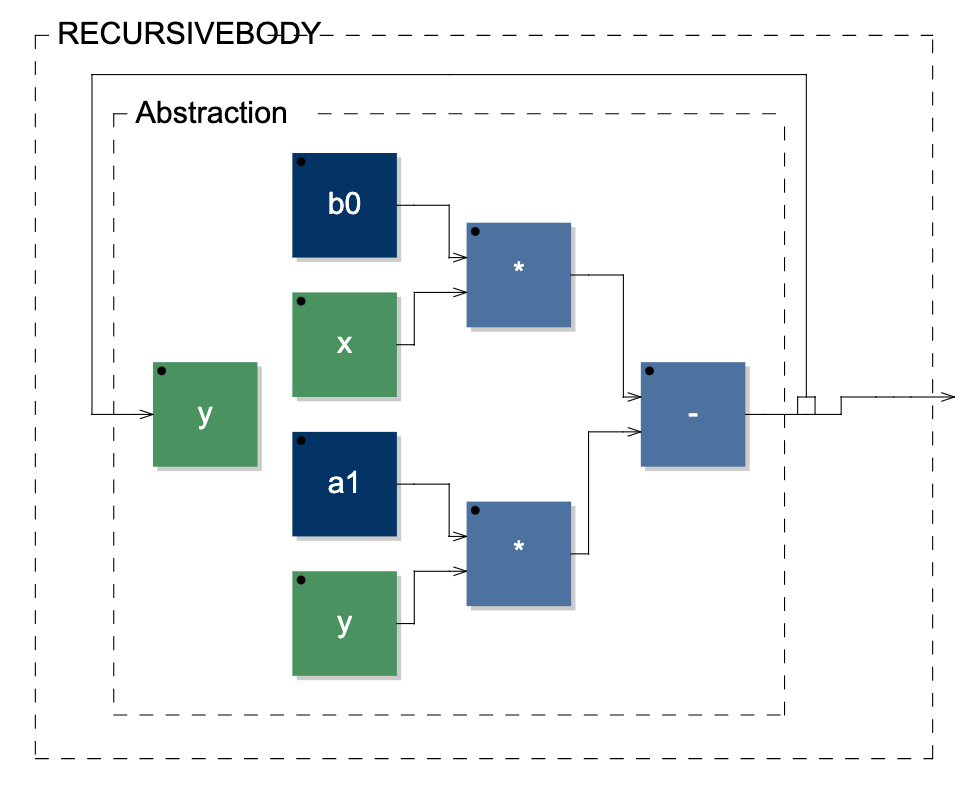Block diagram implemented through the letrec environment.

So far, we have implemented a somewhat elementary circuit. Now, we can try to implement a first-order lowpass filter with zero-delay feedback topology. The circuit below is taken from the book by Zavalishin on virtual analogue filters design.Zavalishin's first-order zero-delay feedback lowpass with resolved topology.

As we can see, the implementation is not as straightforward as the previous case. It can be useful to name several points in the circuit to determine the fundamental signals to compose the whole circuit. Here, we introduce $$G = g/(1+g)$$, $$v$$ as the signal taken after the $$G$$ multiplication, $$s$$ as the state of the system, that is the output of the $$z^-1$$ operator, and $$y$$ as the output of the system. Hence, we have that:

\begin{align*} & v = G(x - s) \\ & y = v + s \\ & s = v + y \\ \end{align*}

If we substitute $$v$$ and $$y$$, then we have that:

\begin{align*} & y = G(x - s) + s \\ & s = 2G(x - s) + s \\ \end{align*}

and we can define two paths, one for the state, the other for the output of the system. Specifically, we can write the paths replacing all occurrences of $$s$$ with a wire, which we will then fill with feedback loops from the state path. It is convenient to define the state first, and the output second, as the tilde operator applies to signals to its left starting from the top: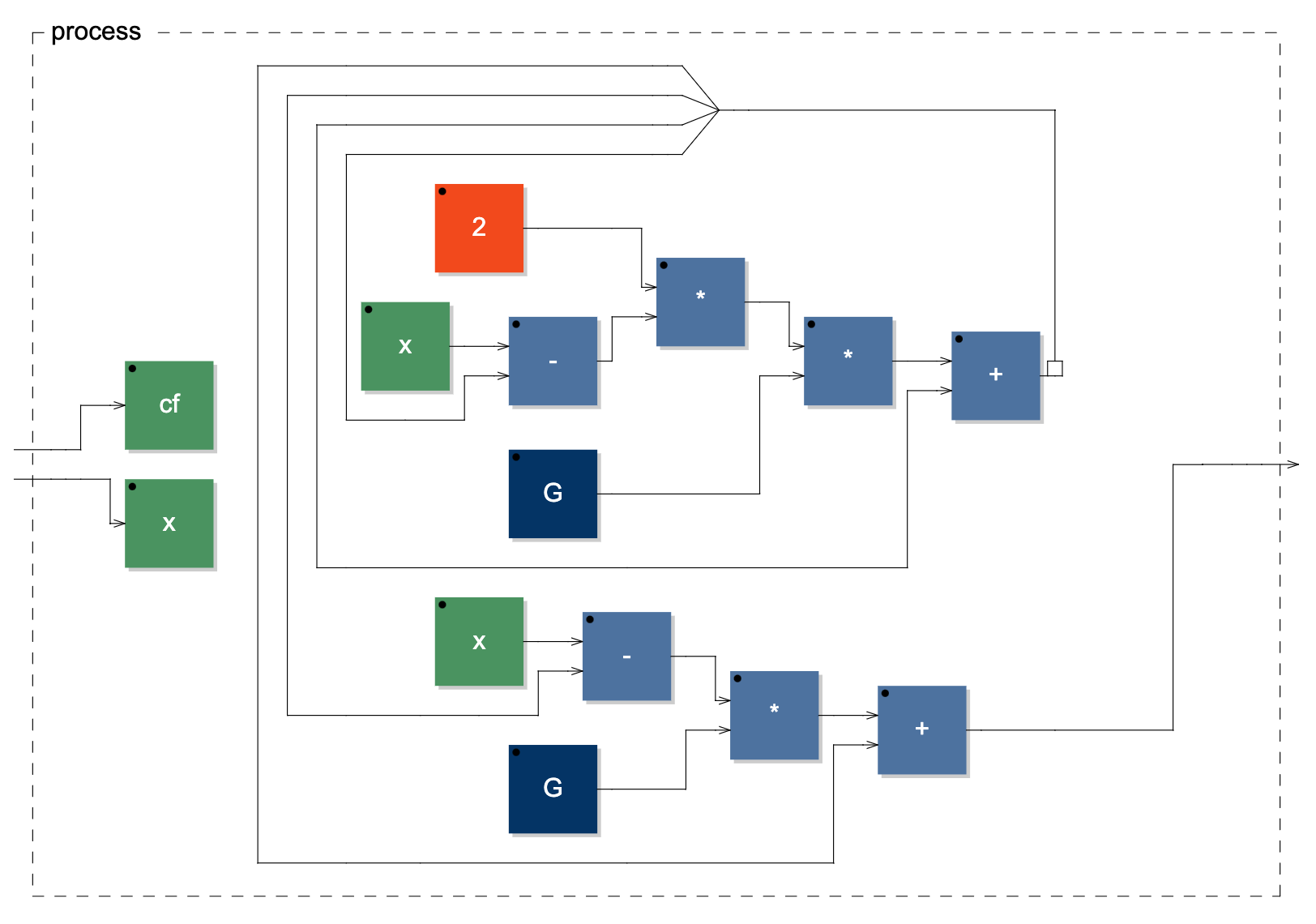Zavalishin's first-order zero-delay feedback lowpass diagram implemented with Faust's basic syntax.

As we can notice, the signal $$G(x - s) + s$$ repeats twice in the diagram. However, Faust’s optimisation will make sure that the signal is computed only once. Still, if we want the diagram to be closer to the original circuit, then we can write the following, copying the signal $$G(x - s)$$ internally to compose the remaining necessary signals: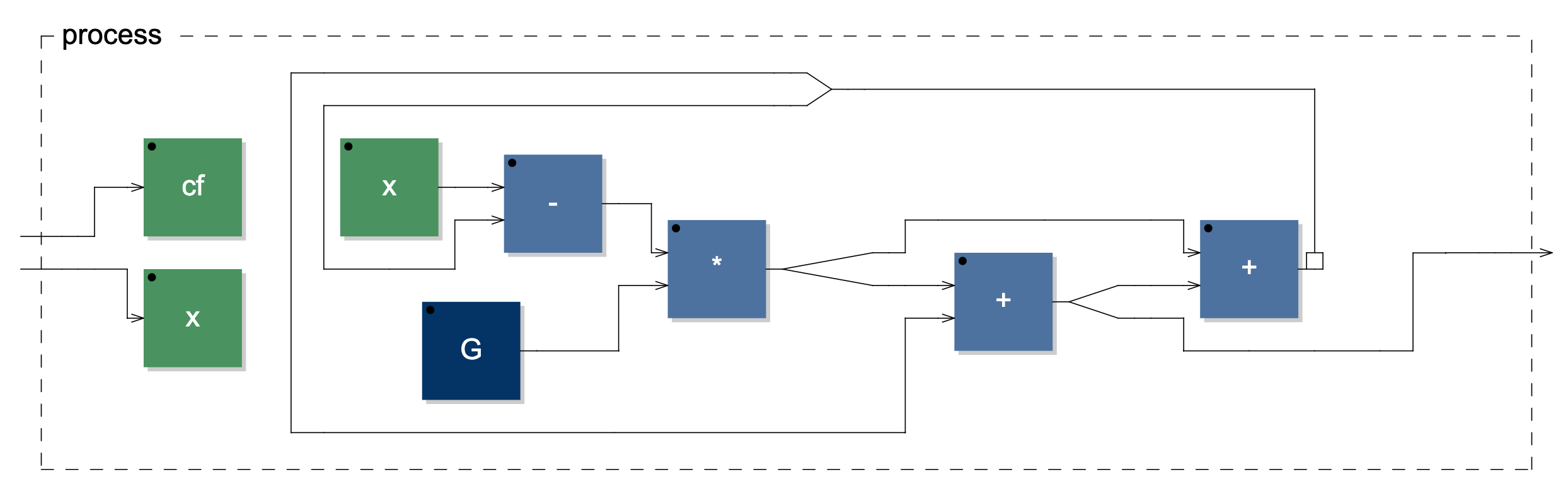Zavalishin's first-order zero-delay feedback lowpass improved diagram.

Now, we can implement the filter using an intermediate function:Zavalishin's first-order zero-delay feedback lowpass diagram implemented through intermediate function.

And finally, we can use the letrec environment for a concise and elegant solution, although the diagram will show some redundancy: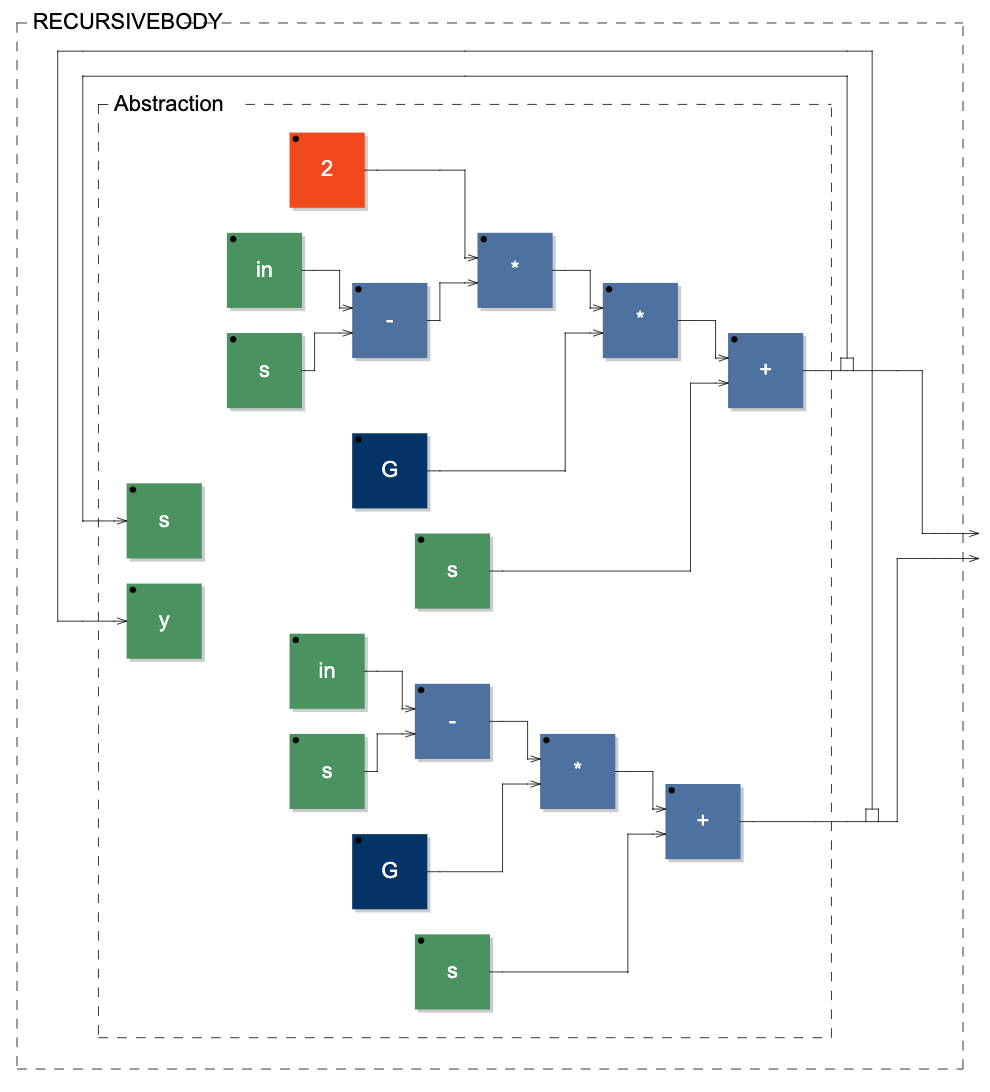Zavalishin's first-order zero-delay feedback lowpass diagram generated through the letrec environment.

For the last example, we will implement Martin Vicanek’s beautiful quadrature oscillator, a recursive self-oscillating system with two states. See the circuit below.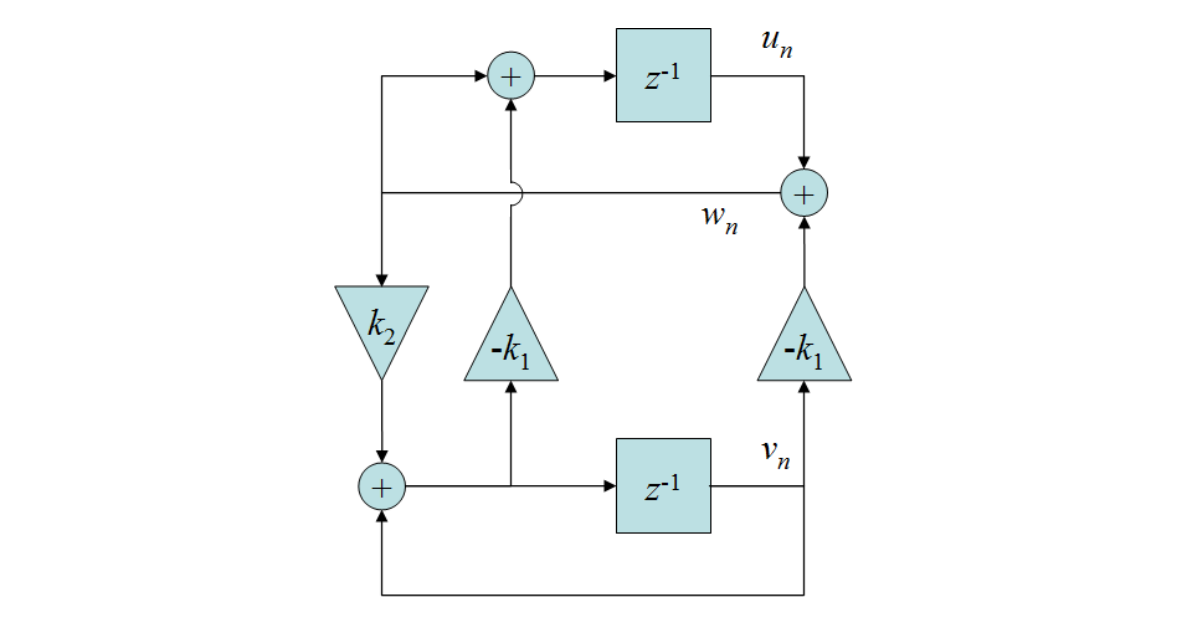Here, we have a feedback system with two cross-coupled states. Hence, it is not as straightforward as with systems having only one state, for we must send each state back to the appropriate inputs. In this system, we need to define two state paths, which correspond to the two outputs of the system. Similarly to what we did earlier, we can define the states by composing the paths with the signals feeding into the $$z^-1$$ operators. Thus, the two states $$u_n$$ and $$v_n$$ are defined as follows:

\begin{align*} & u_n = w_n - k_1(v_n + k_2 \cdot w_n) \\ & v_n = v_n + k_2 \cdot w_n \\ & w_n = u_n - k_1 \cdot v_n \\ \end{align*}

If we substitute $$w_n$$, we have that:

\begin{align*} & u_n = u_n - k_1 \cdot v_n - k_1(v_n + k_2(u_n - k_1 \cdot v_n)) \\ & v_n = v_n + k_2(u_n - k_1 \cdot v_n) \\ \end{align*}

To start with, using basic syntax, we will simply put a wire wherever a state is fed back without distinguishing between $$u_n$$ or $$v_n$$:

This will lead to the following network, where the external inputs are feedback paths that need to be matched with the corresponding states.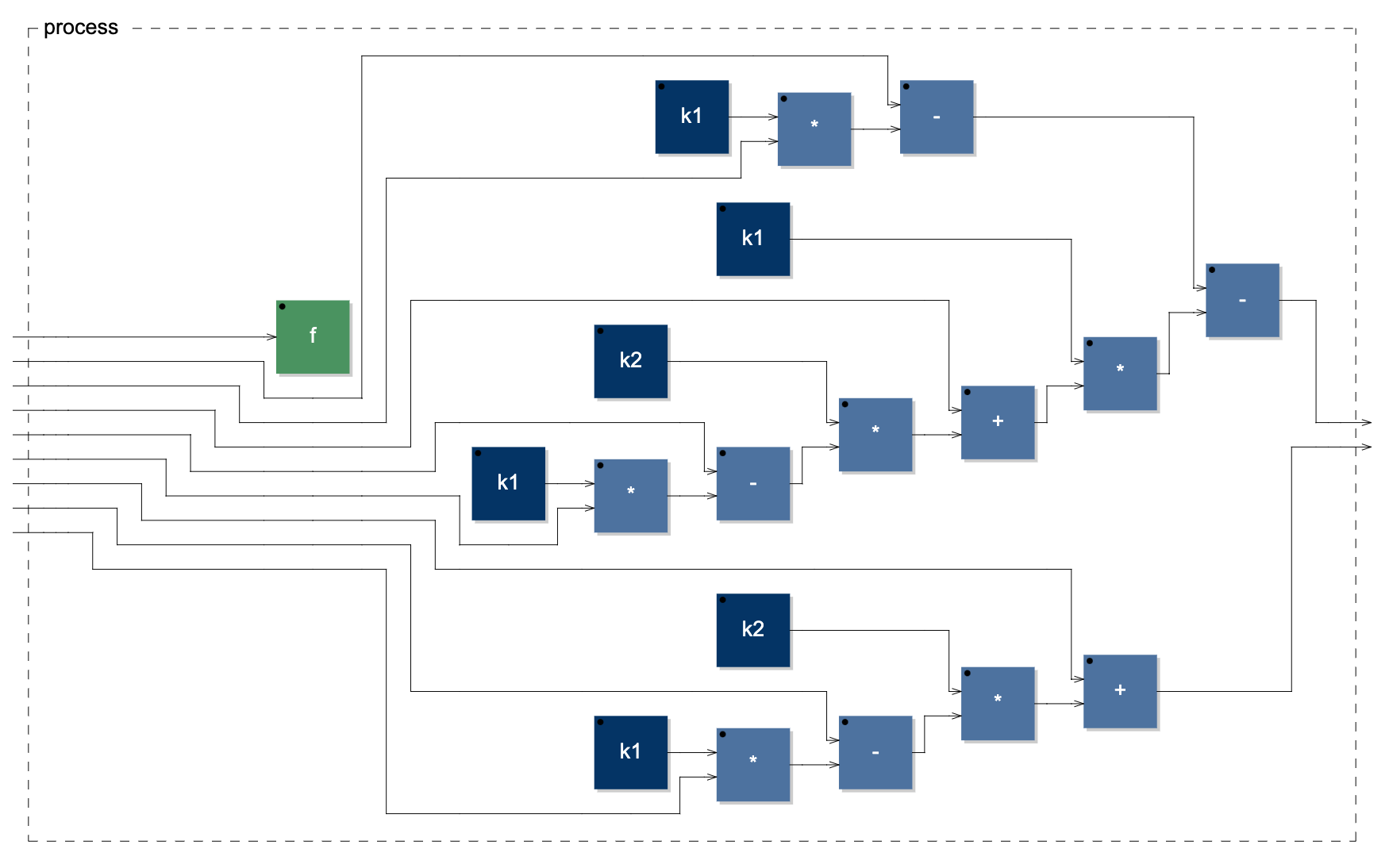Intermediate implementation stage of Vicanek's oscillator.

At this point, and without worrying about redundancy in the resulting diagram, the easiest thing to do is to send the two states to the feedback path and then copy and route them accordingly. We can do so using the “route” primitive, which we can call specifying the number of inputs, the number of outputs, and a set of input-output pairs to route the signals. Furthermore, we will also add a one-sample impulse to the $$u_n$$ state as its initial condition must be 1.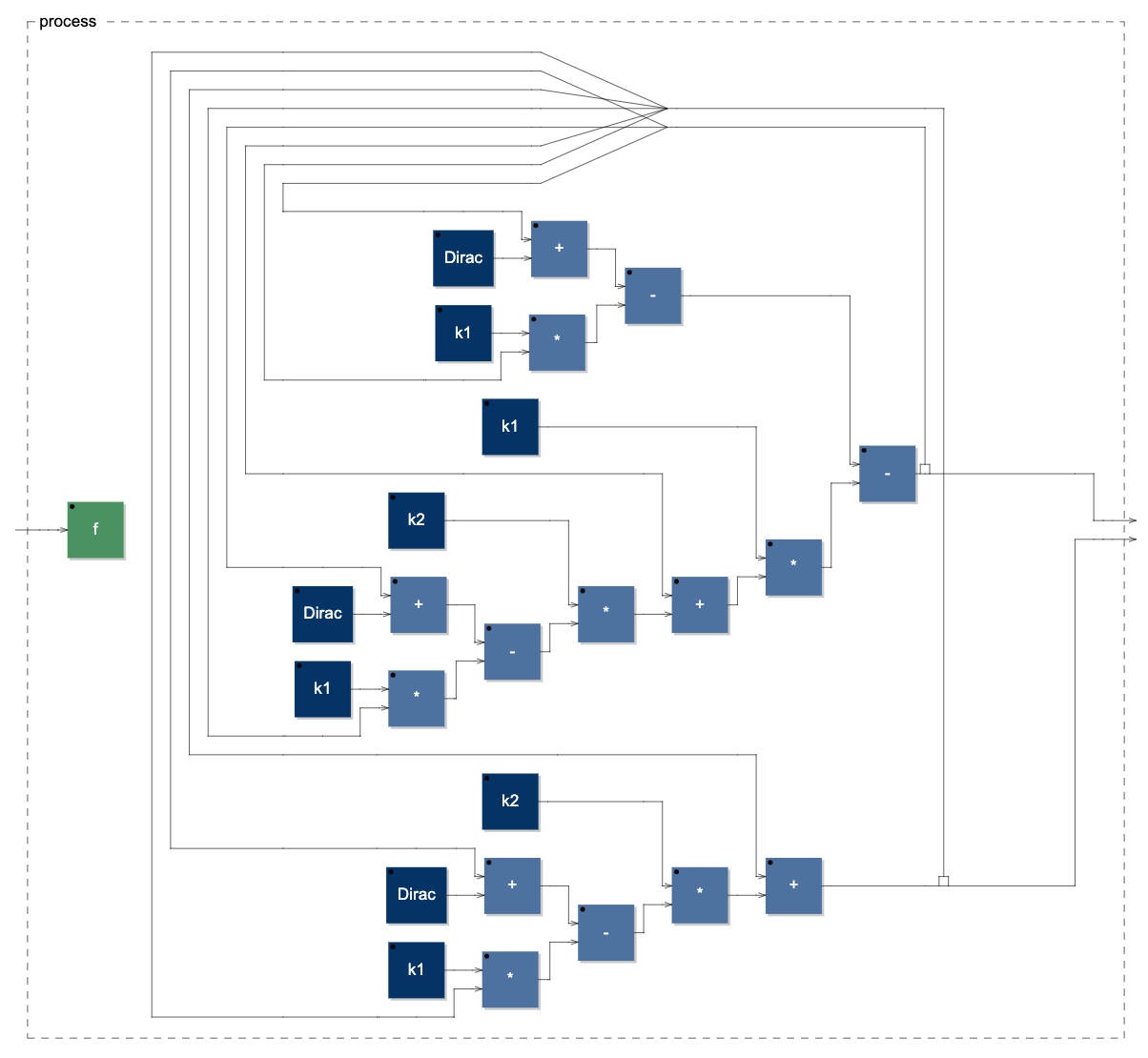Vicanek's oscillator diagram implemented through Faust's basic syntax.

Next, we can see how to implement the oscillator using the second approach. It should be clear now: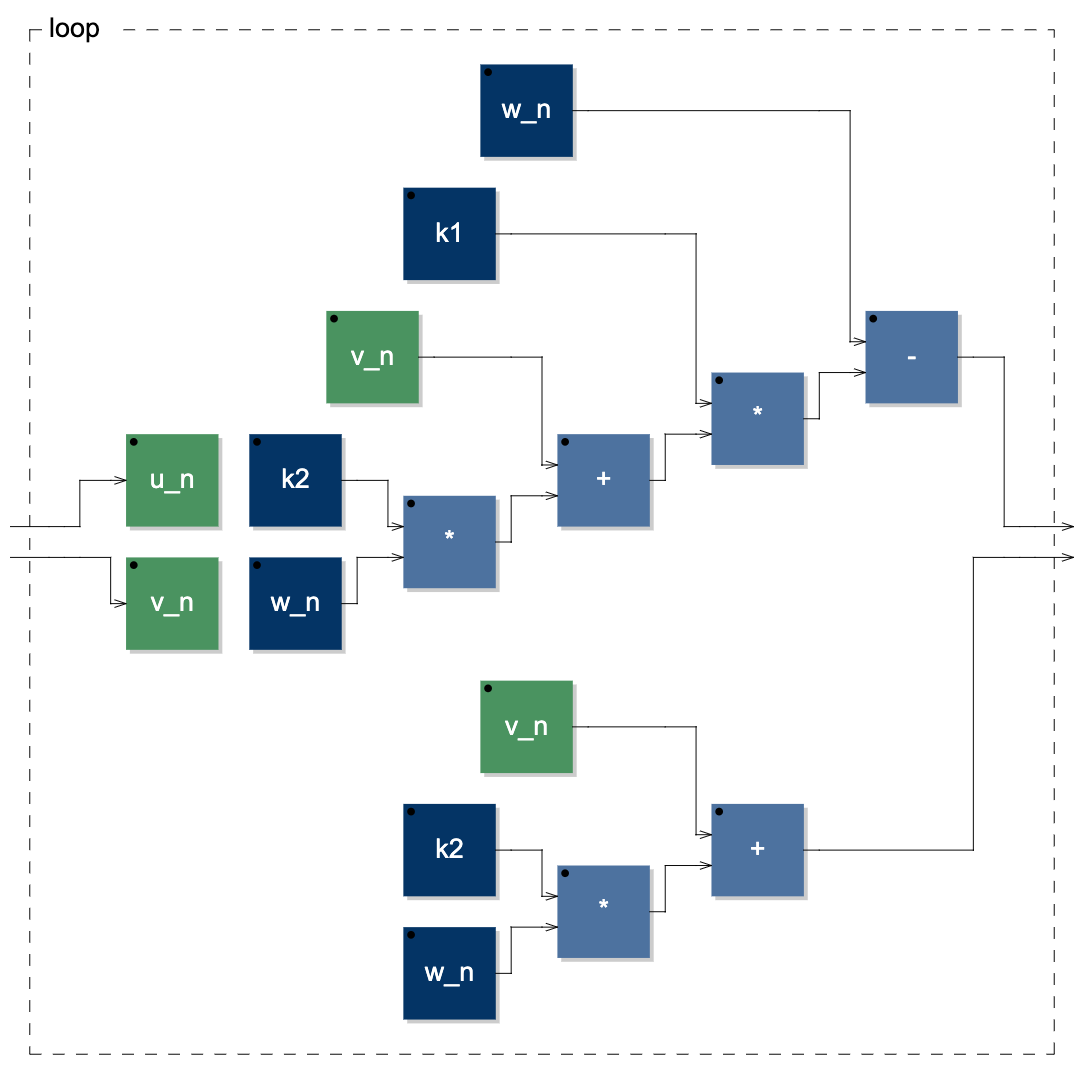Vicanek's oscillator diagram implemented through an intermediate function.

Lastly, we can see how to implement the system using letrec: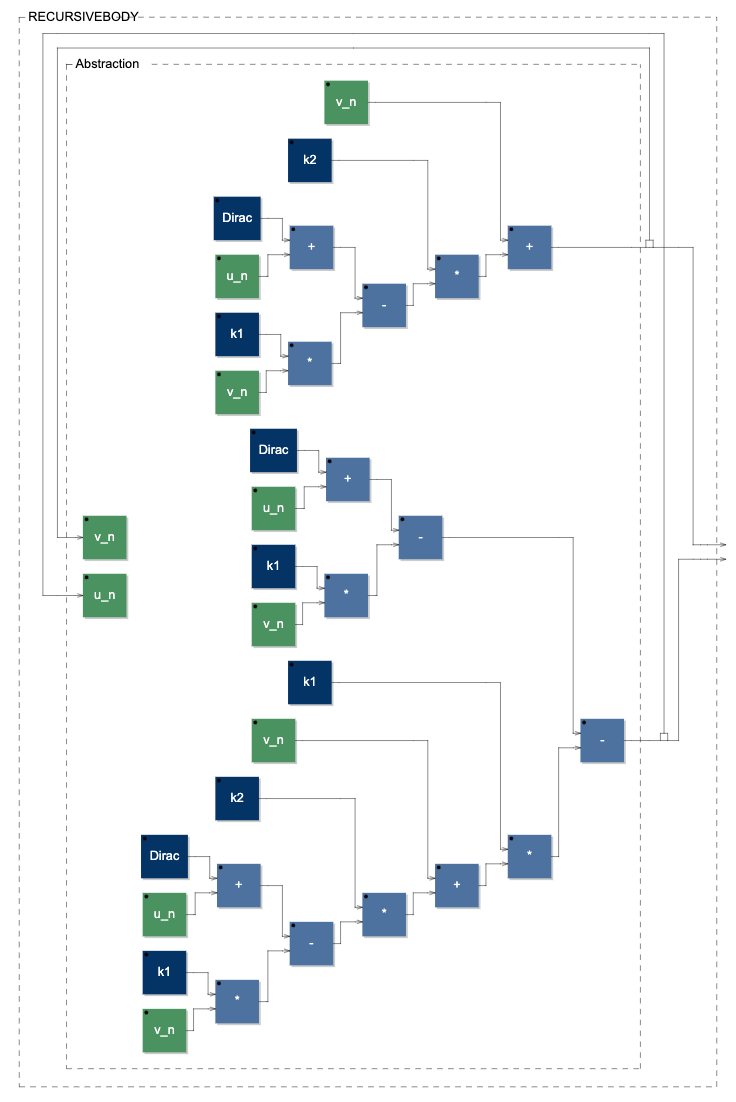Vicanek's oscillator diagram implemented through the letrec environment.

Overall, it seems that the with and letrec environments are best to work with. Particularly the with environment with auixiliary function, it allows to define temporary or intermediate signals as we did for the quadrature oscillator. Using letrec, instead, that would not be possible as it would introduce a delay in the auxiliary path. The basic syntax, though, is still useful when we want to generate diagrams showing the entire network topology.

]]>
A few more thoughts on zero-crossing granular processing2020-04-16T15:42:14+00:002020-04-16T15:42:14+00:00www.dariosanfilippo.com/blog/2020/a-few-more-thoughts-on-zero-crossing-granularAs it often happens, you start the implementation of an algorithm that initially seems to be straightforward and eventually a number of challenges emerge, making the implementation more difficult and intriguing.

This is what happened to me with the implementation of a windowless granulator based on zero-crossing (ZC) detection and delay lines. I will briefly summarise the main points below, depicting what seems to be a fairly advanced stage of the implementation that has given good results.

• The content of a delay line of size L can be static: such delay line should be filled with the output of a feedback loop of period L that is, in turn, filled with an input signal x. The gains of the input signal and feedback path are mutually exclusive to transition from live to looped inputs.
• For a given pitch shift and time transposition factors, the complement of such factors determines the slopes of line functions that are used, respectively, to modulate the delay and to offset the delay start.
• For proper continuity in the signal, both the end of the current grain and the beginning of the next one must at a ZC.
• For proper continuity in the signal, both the derivatives at the end of the current grain and at the beginning of the next one must have the same sign.
• For proper continuity in the signal, the position of the next grain must be corrected to avoid the repetition of samples – two samples at a ZC.
• The sample position correction can be obtained as the ratio between the derivatives at the end and the beginning of grains. This prevents the derivative of the signal to suddenly change in sign when transitioning from a high-rate grain to a slower-rate one.
• Negative pitch transposition factors result in grains being read backwards, which in turn result in a change of sign in the derivative of the signal. This must be taken into account to select the next grains with the right derivative sign, and the right direction for the sample position correction – either forward or backward.
]]>
Granulation without windowing using delay lines and zero-crossing detection2019-05-11T13:21:03+00:002019-05-11T13:21:03+00:00www.dariosanfilippo.com/blog/2019/granulation-without-windowing-using-delay-linesThis post is a follow-up to the previous post on granulators using zero-crossing (ZC) detection. Some information has already been discussed, but more details have been added here.

The main idea behind a ZC granulator is that grains start and end at a ZC. ZC can be detected by observing the sign of the product between the current sample and the previous one:

zc[n] = {1, if x[n]x[n - 1] < 0; 0, otherwise },

where x[n] is the input of the function. If we have a fixed audio source on a table, we can then scan the table and store all the ZC positions in an array of as many elements as the ZC occurrences so that they can be recalled at a later time. But signals can be irregular, and so can be ZC. If we want both the start and end of grains to be at a ZC, it means that the duration of each grain is variable and dependent on the signal itself.

The fundamental condition for a sequence of grains of duration D without discontinuities is that each successive grain should be triggered after the time D has passed, at the first ZC occurrence. It means that the output of the granulator must be continuously inspected to detect a ZC, and such information must be sent back into the section that generates each grain. It is the minimum requirement for a continuous stream without discontinuities, although harmonics, noise, and aliasing may be introduced without further adjustments.

One crucial aspect is to have consistency between the direction of the signals at the end of grain and that at the beginning of the successive one. The direction of a signal is given by the sign of its first derivative:

direction_up[n] = {1, if x[n] - x[n - 1] > 0; 0, otherwise};
direction_down[n] = {1, if x[n] - x[n - 1] < 0; 0, otherwise}.

The ZC positions of the input that we want to process can then be stored into two different arrays: one for the ZC occurring in ascending signals, the other for ZC in descending signals. Similarly, the output of the granulator can be analysed for both direction and ZC so that the position of the next grain is selected from the corresponding set of ZC indexes.

Another improvement to have a smoother transition between grains is to start a grain from the successive sample than the one at the ZC position. Presumably, if both the end and beginning of grains are at a ZC position, they might be at a very close value which might result in the repetition of two samples. By skipping one sample at the beginning of each grain, there is a better continuity and smoothness in the resulting signal.

If the input signal is not fixed and we are using a circular buffer (CB) to update it continuously, then we can use two CB of the same size to store the ZC indexes of ascending and descending signals. To do so, we sample-and-hold (SAH) the indexes at which a ZC is detected so that any recalled position in the ZC buffers corresponds to a ZC position in the input buffer. A SAH unit has two inputs: c[n], a Boolean value, controls the sampling process; x[n] is the signal to be sampled:

SAH[n]  = {x[n], if c[n] = 1; SAH[n - 1], if c[n] = 0}.

If the size of the CB is S, then the writing index, i[n], cycles through integers from 0 to S-1. i[n] is the signal that we want to store in the ZC CB, whereas the conditions to trigger the SAH in ascending and descending signals are, respectively:

zc[n] AND direction_up[n],
zc[n] AND direction_down[n].

In Faust, tables do not implement fractional indexes and are not ideal for pitch transposition; fractional delay lines are often used for live granular processing with pitch transposition. In the case of tables, recalling a ZC index is rather straightforward, and it is enough to read the input buffer at that position. With delay lines, since we move around the buffer by setting a delay relative to the position of the writing index, a few more steps are necessary.

In delay lines of length L samples, the writing index, i[n], cycles through integers from 0 to L - 1. This index is what we sample-and-hold when the ZC are detected and represents the time after which, relative to the beginning of the process, a ZC has occurred. It is essentially a time offset, and we can recall a ZC that has occurred at previous time P by setting the delay to i[n] - P. Of course, if P is greater than the current index i[n], then the negative value should be wrapped around the [0;L] range. A general wrapping function has the following form:

wrap[n] = fractional((x[n] - min) / (max - min))(max - min) + min.

By simply reorganising the input signal by arranging grains at different ZC positions in the buffer, we have a granulator without transposition. At this point, the pitch transposition of each grain can be implemented as a delay shift starting from the selected ZC position. If the desired grain rate is R, which determines the grain duration 1 / R, then the delay shift for a given pitch factor (PF) can be calculated as follows:

(1 - PF)(1 / R)(line)SR,

where SR is the samplerate and line is a signal that grows from 0 to 1 in 1 / R seconds. A line can be implemented as follows:

y[n] = R / SR + y[n - 1].

In the image below, we can see the spectrum of a 1kHz sine wave reconstructed through grains randomly selected over the whole buffer at a rate of 100 grains per second, and pitch-shifted of a factor of 3. Of course, the process does introduce some noise but it shows that it is correct. (It may not be clear from the image but the peak is centred at 3kHz; the SNR is about 60dB.)

Currently, I am particularly satisfied with the noisy textures generated by this algorithm, as the windowless design has a particular sharpness even at lower grain rates, which I could not perceive with the standard design.

]]>
Early experiments with granulators and zero-crossing detection2019-04-21T17:04:37+00:002019-04-21T17:04:37+00:00www.dariosanfilippo.com/blog/2019/early-experiments-with-granulators-andDiscontinuities in granulators are commonly handled using windowing functions, though they sometimes create a recognisable sound and I was looking for alternative techniques.

Zero-crossing (ZC) detection can be deployed to avoid discontinuities between grains.

In Pure Data, I had already done some experiments last year with this technique using audio samples and static buffers. The sounds were nice and crisp though a proper implementation wasn’t possible because of some limitations in PD.

I am now implementing my new systems in Faust and the image shows the Faust diagram of the main unit in the granulator.

For this implementation, I’m using Faust’s read-write tables as circular buffers for the read and write indexes can be piloted through signals and there are no issues with synchronisation among several buffers.

This algorithm uses three circular buffers. One buffer is used to store the processed signal; the other two buffers are used to store the index position at which a ZC has been detected in ascending or descending signals.

“Frame“ linearly cycles through integers starting from 0 to read the samples in the buffer. At each new cycle, a new ZC position is sampled and used as a starting position for the grain. The position is then fed back into “frame“ to calculate the end position for that grain, which is the ZC position closest to the desired length of the grains. To keep consistency for ascending and descending signals, the output of the granulator is tracked down with a differentiator to calculate the slopeness that is sent back into the main module, in the “sel_zc“ object, so that the next position is sampled from the right set of ZCs.

Quite happy of how it sounds and the next step might be to implement the algorithm with variable delay lines to make grain transposition possible, although synchronisation might not be as easy.

]]>
implementing digital deterioration by increasingly2018-11-30T12:13:32+00:002018-11-30T12:13:32+00:00www.dariosanfilippo.com/blog/2018/implementing-digital-deterioration-by-increasingly

## Implementing digital deterioration by increasingly unstable nonlinear feedback networks: an exploration of phase transitions in dynamical systems

It’s been three months since I moved to Vienna and it feels like I moved here three days ago. The city is great, just as I remembered it, and I like the people I’m working with.

Rotting Sounds, whose project manager is Thomas Grill, is an artistic research project investigating the idea of temporal deterioration of digital audio. Thomas invited me to contribute to the project after I told him I was gonna move to Vienna. After a few meetings and discussions, I came up with the idea of working with feedback systems that progressively become unstable to structurally and conceptually render the idea of digital deterioration.

The main purpose is to implement a set of relatively small networks with different topologies, feedback matrixes, and nonlinearities to microscopically explore the phase transitions of the systems by means of adaptive behaviours. The works will be exhibited at the Mold Museum of Sounds starting from April and the deterioration process will take place over a period of weeks.

Pure Data is the software that I normally use for my works, but the programming environment used for these networks is the Faust language, for double precision in the DSP calculations is a requirement given the very long lifespan that these networks need to achieve full deterioration.

The periods of the feedback loops in the networks, which may be chosen as prime, co-prime, or near-integer ratios depending on whether more or less spectral peak overlappings are desired, will be affected by one or more features of the environment where the works will be running.

The nonlinear functions will be a set of bounded saturators. These functions work in a way such that the waveshaping is directly proportional to the amplitude of the input signal, which can be used as a deterioration process for progressively growing signals.

Networks will start from the condition of marginal stability, that is a configuration of the nonlinear functions and feedback coefficients so that a constant energy stream is produced by a Dirac impulse. Over a time span of one or more weeks, the feedback coefficients of the networks will be increasing from the stability threshold to an arbitrarily chosen value outside of the stability range. The systems will soon become self-oscillating but the limiting effect of the saturators will prevent them from growing infinitely.

As the feedback coefficients increase, the input of the nonlinear functions will grow too, resulting in a stronger deterioration which in turn will produce richer spectra. With more frequency components, there will also be more interactions between signals and instabilities.

The output of the system is the result of recursively combined intermodulation phenomena – both at formal (beats) and timbral (sidebands) time scales – together with the iterated nonlinearities inherent in the DSP structure.

Phase transitions are particularly interesting and nontrivial states of dynamical systems, and the most profound aesthetic aspect of this work is the time-stretched exploration of such areas while going through different degrees of instability. This microscopic inspection will be realised by implementing an adaptive behaviour that affects the growth rate of the feedback coefficients. Specifically, the detection of a phase transition will slow down the growth of the coefficients, while the detection of a stable state will increase it.

]]>

About three years ago I was writing a goodbye post to Vienna; I was leaving the city to start my PhD in Edinburgh.

After all this time, I can look back and be happy about that choice: Edinburgh is certainly not my favourite city but I’ve met some really nice people and we’ve done a very good job for my PhD together with my supervisors. My research has produced some very convincing results and I am now in its final state, writing the thesis, recording the performance projects for the portfolio, as well as putting together a library with some of the software that I have developed.

Today I’m writing a goodbye post to Edinburgh after I just moved back to Vienna, a city that I love very much. It is hard to tell how long I will stay here and how things will turn after I finish my PhD, but it feels great to be back and I am currently working on two interesting research projects together with two of my best friends here. The projects both involve 1-bit digital audio but one focuses on digital deterioration, while the other focuses on FPGAs and delta-sigma modulation. I will mainly be working on some pieces for the first one and I will investigate adaptive recursive Boolean networks for the second.

Exciting times ahead and the sun is shining.

]]>
Lectures, workshops and concert at the Sibelius Academy during 16th-23rd of April2018-03-23T13:55:14+00:002018-03-23T13:55:14+00:00www.dariosanfilippo.com/blog/2018/lectures-workshops-and-concert-at-the-sibeliusI’ll be a guest at the Sibelius Academy in Helsinki during 16th-23rd of April as part of a Staff Erasmus programme. I will present my research and we will have workshops and discussions about music complex adaptive systems and some DSP techniques related to the field. I will also give a concert with two of my works on the 18th of April. I hope to see you there.

]]>
Brief remarks on an algorithm for the estimation of the lowest partial of a signal (and, consequently, its highest partial and, consequently, its bandwidth)2018-01-29T23:37:22+00:002018-01-29T23:37:22+00:00www.dariosanfilippo.com/blog/2018/brief-remarks-on-an-algorithm-for-the-estimationA couple of years ago I implemented a fairly robust and certainly CPU-friendly algorithm for the time-domain estimation of the spectral tendency. It works by using a crossover to divide the input spectrum into two parts whose energy is measured through the RMS. The imbalance between the two spectra is what creates a negative feedback loop by shifting the cutoff of the crossover towards the predominant side. As a result, the system quickly starts to (minimally) oscillate around the point of equal energy providing a rather accurate estimation of the spectral tendency.

One simple extension to this algorithm which I have recently implemented was to put a lowpass filter within the feedback loop, on top of the chain. Let’s remember that the spectral energy imbalance pushes the cutoff of the crossover towards the predominant side. What happens if that imbalance is used to pilot the cutoff of the lowpass filter too? The result is a positive feedback loop for the lowpass filter will weaken the upper part of the spectrum and the imbalance will be pushing towards the lower part even further. This recursive process of spectral attenuation, from high to low components, will finally end when there are no components left on the lower side of the spectrum as the negative feedback loop will now be oscillating around the equal energy point, which is the frequency of the lowest partial. The same principle can be used to implement a system which removes all frequency components up to the last one in the upper part of the spectrum, and the combination of the two can be used as an estimation of the bandwidth of a signal.

The problem with this kind of algorithms is that the filters need to be very selective. For the spectral tendency estimator, I am using 1-pole-1-zero highpass and lowpass filters for the crossover and that seems to be a good compromise: considering that the energy difference is what matters, the fact that the filters have large transition bandwidths is not a problem as they will overlap and counterbalance each other out. With the algorithm discussed here, the quality of the lowpass or highpass needs to be very high for removing the components otherwise the non-attenuated parts will affect the accuracy of the result. Namely, for this algorithm, I am using four cascaded 1-pole-1-zero filters and I am having fairly acceptable results for signals whose lowest components are around Nyquist/2. Above that, there is less resolution and the results are compromised.

One way to improve the algorithm could be to use elliptic filters. These have a very narrow transition band at low orders but also some fairly large ripples in the passing band, though that would not compromise the correct behaviour of the algorithm and a stronger attenuation of the components would hopefully give good results throughout the whole spectrum.

Below you can see a simplified diagram of the system: some parts necessary to prevent it from entering attractors have been omitted.

]]>